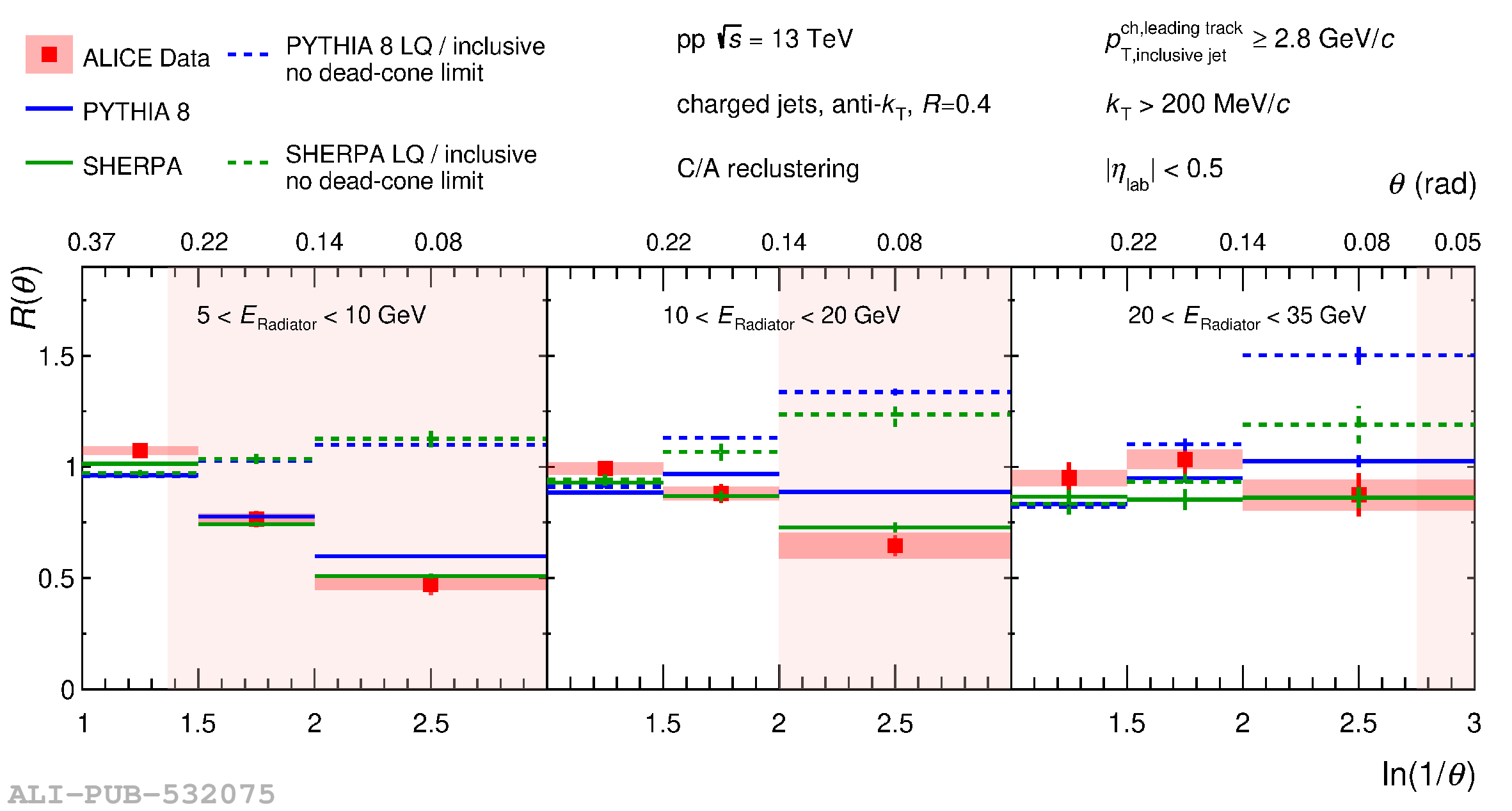# Figure 2

 Ratios of splitting angle probability distributions. The ratios of the splitting-angle probability distributions for D$^{0}$-meson tagged jets to inclusive jets, $R(\theta)$, measured in proton--proton collisions at $\sqrt{s}=13$ TeV, are shown for $5 < E_{\rm{Radiator}} < 10$ GeV (left panel), $10 < E_{\rm{Radiator}} < 20$ GeV (middle panel) and $20 < E_{\rm{Radiator}} < 35$ GeV (right panel). The data are compared with PYTHIA v.8 and SHERPA simulations, including the no dead-cone limit given by the ratio of the angular distributions for light-quark jets (LQ) to inclusive jets. The pink shaded areas correspond to the angles within which emissions are suppressed by the dead-cone effect, assuming a charm-quark mass of $1.275$ GeV/$\it{c}^{2}$.